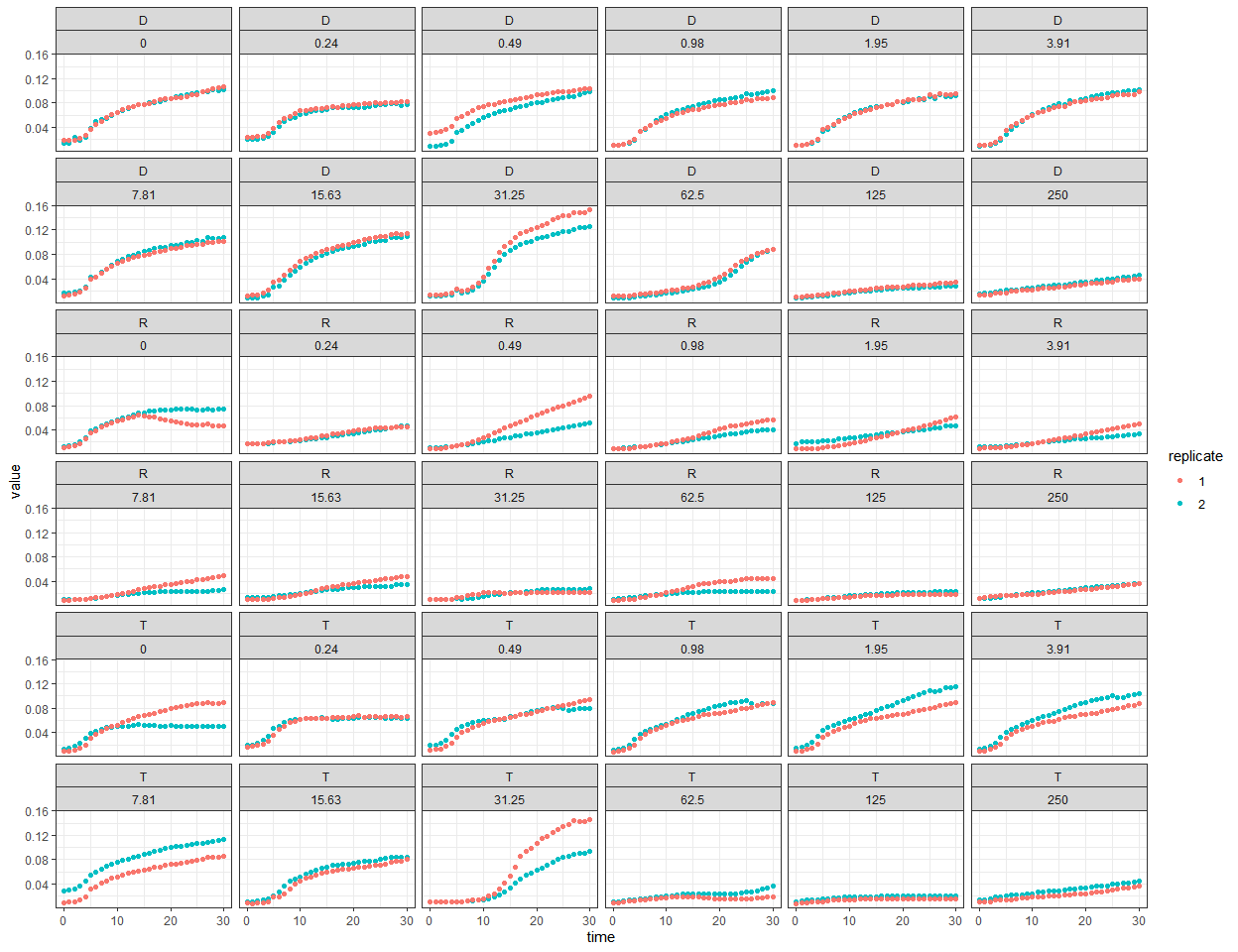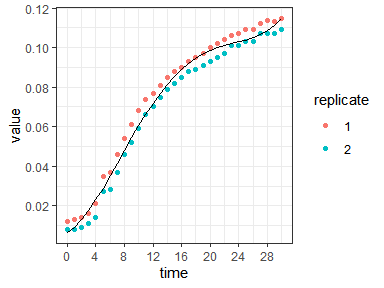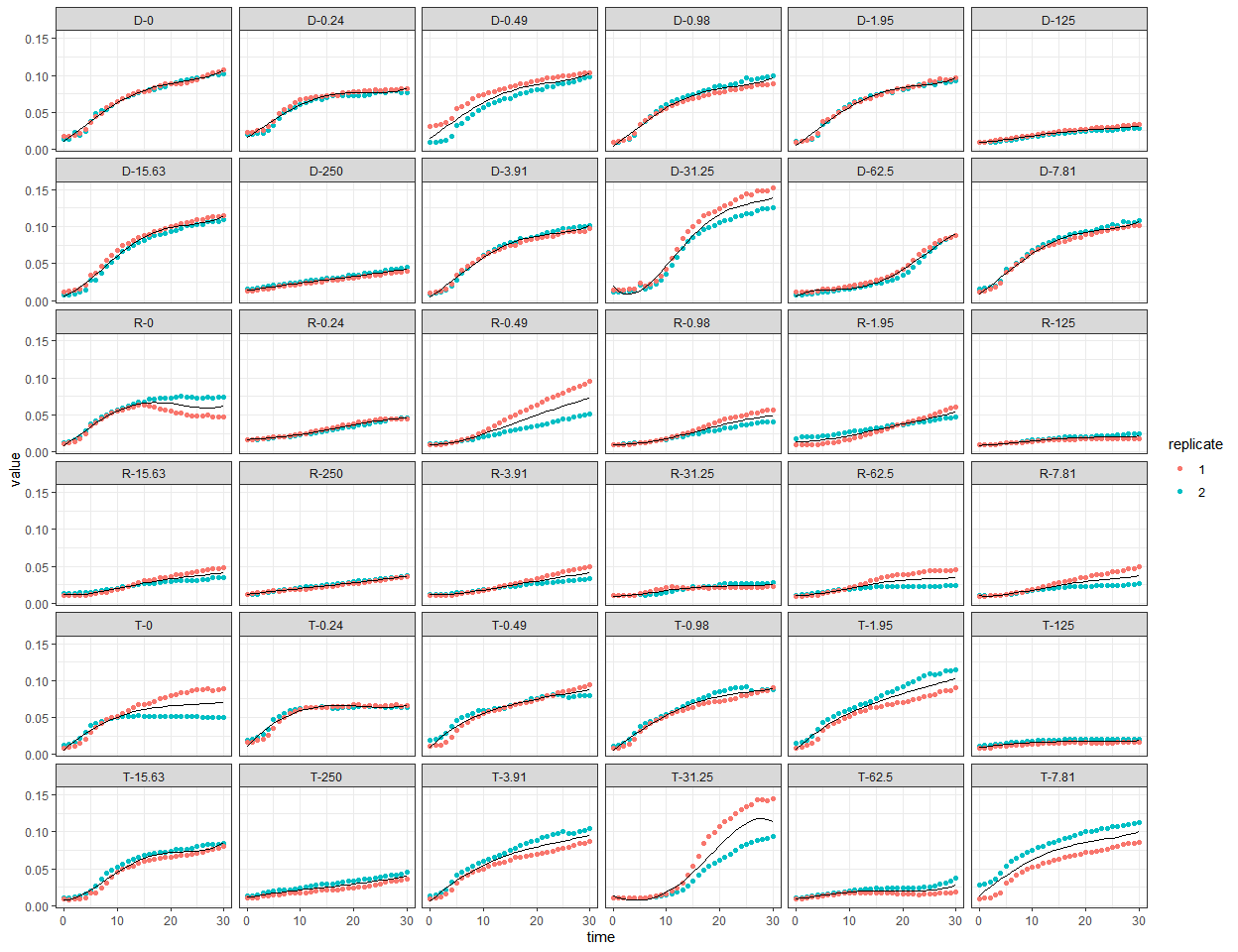# Using ipolygrowth for bacterial growth estimation

## Introduction

The ipolygrowth package calculates bacterial growth curve parameters using 4th degree polynomial functions. Polynomial growth curves are estimated from time series data from a single biological sample or multiple samples. Technical replicates within biological samples are allowed.

In this vignette, we will demonstrate examples with both single and multiple samples as input data. To use this package, we need to start with installing and loading the ipolygrowth package.

# install.packages("ipolygrowth")
library(ipolygrowth)
library(dplyr)
library(ggplot2)
library(kableExtra) # for RMarkdown table output

### Example data

We will use the bacterial growth data from the R package growthrates for demonstration. This data contains growth data of 3 bacteria strains and 12 antibiotics tetracycline concentration levels. Each strain-concentration combination has 2 replicates. In each replicate, the growth of bacteria is measured 31 times (i.e. 31 time points in the time series). For our demonstration, we consider each strain-concentration combination as a sample (i.e. a biological replicate) and each replicate as a technical replicate.

Let’s load the data and check its structure.

df.gr <- growthrates::bactgrowth
str(df.gr)
#> 'data.frame':    2232 obs. of  5 variables:
#>  $strain : Factor w/ 3 levels "D","R","T": 3 3 3 3 3 3 3 3 3 3 ... #>$ replicate: int  2 2 2 2 2 2 2 2 2 2 ...
#>  $conc : num 0 0 0 0 0 0 0 0 0 0 ... #>$ time     : int  0 1 2 3 4 5 6 7 8 9 ...
#>  $value : num 0.013 0.014 0.017 0.022 0.03 0.039 0.042 0.045 0.048 0.049 ... We can also take a look at the first few rows of the data. head(df.gr) #> strain replicate conc time value #> 1 T 2 0 0 0.013 #> 2 T 2 0 1 0.014 #> 3 T 2 0 2 0.017 #> 4 T 2 0 3 0.022 #> 5 T 2 0 4 0.030 #> 6 T 2 0 5 0.039 Let’s plot the data. ggplot()+ geom_point(data = df.gr, aes(x = time, y = value, color = factor(replicate)))+ facet_wrap(~ strain + conc)+ labs(color = "replicate")+ theme_bw()### Requirement of data structure for ipolygrowth The ipolygrowth functions require the input data to be in long format with a time variable and a dependent variable (y) as the measure of growth in the input data. Both variables need to be numeric. Time points in the time series can be any length (uneven and different spacing allowed, even between technical replicates), as long as the time scale is measured uniformly (same units used) across all samples. ## When you have a single sample We will use the ipg_singlesample() function to calculate growth curve parameters for one sample. Since the growthrates data contains 36 strain-concentration combinations, we will use data from donor (D) with concentration 15.63, to represent two replicates from a single sample. We will take out the variables strain and conc from the data frame to avoid confusion. df.singlesample <- df.gr %>% filter(strain == "D", conc == 15.63) %>% select(-strain, -conc) str(df.singlesample) #> 'data.frame': 62 obs. of 3 variables: #>$ replicate: int  2 2 2 2 2 2 2 2 2 2 ...
#>  $time : int 0 1 2 3 4 5 6 7 8 9 ... #>$ value    : num  0.008 0.008 0.009 0.011 0.014 0.027 0.028 0.037 0.046 0.052 ...
table(df.singlesample$replicate, df.singlesample$time)
#>
#>     0 1 2 3 4 5 6 7 8 9 10 11 12 13 14 15 16 17 18 19 20 21 22 23 24 25 26 27
#>   1 1 1 1 1 1 1 1 1 1 1  1  1  1  1  1  1  1  1  1  1  1  1  1  1  1  1  1  1
#>   2 1 1 1 1 1 1 1 1 1 1  1  1  1  1  1  1  1  1  1  1  1  1  1  1  1  1  1  1
#>
#>     28 29 30
#>   1  1  1  1
#>   2  1  1  1

Now we specify the data frame name, time variable, and y variable in the ipg_singlesample() function and save the results. The output of ipg_singlesample() contains a table of calculated growth curve parameters, the polynomial model deriving the calculated parameters, a table of $$\beta$$ coefficients, and a table of fitted values with time. All components can be viewed by calling from the list output. Here, we show the table of calculated growth curve parameters.

out.singlesample <- ipg_singlesample(data = df.singlesample, time.name = "time", y.name = "value")
#> max y time is equal to the largest value of "time"

out.singlesample$estimates %>% kable %>% kable_styling("striped", full_width = F) # table formatting for rmarkdown peak growth rate peak growth time doubling time lag time max y max y time 0.0064197 7.246653 107.972 1.515281 0.1149354 30 We can use the fitted values and the original data to plot our results. ggplot()+ geom_point(data = df.singlesample, aes(x = time, y = value, color = factor(replicate)))+ geom_line(data = out.singlesample$fitted, aes(x = time, y = fit))+
labs(color = "replicate")+
scale_x_continuous(n.breaks = 10)+
scale_y_continuous(n.breaks = 7)+
theme_bw()### Altering epsilon for the maximum in y

Notice the printed message “max y time is equal to the largest value of”time”” after ipg_singlesample() is called. This message appears when max y time is equal to the largest observed time point in the sample and when the search algorithm to identify max y time did not converge. Usually, this indicates the growth curve did not reach an asymptote. If this message is printed, we recommend to plot the data from the sample to ensure the calculated max y time is appropriate for your data.

epsilon is a tuning parameter to change the threshold in the calculation of max y time. epsilon must be between 0 and 1, and a small value is recommended as it represents the convergence threshold as a fraction of the range of the dependent variable. The default value is 0.2%. The table below shows how the max y time is affected when epsilon is set to 1%. For this data, a reasonable value of epsilon is 1% as it corresponds to max growth at the end of the first growth phase.

out.singlesample2 <- ipg_singlesample(data = df.singlesample, time.name = "time", y.name = "value", epsilon = 1/100)
out.singlesample2$estimates %>% kable %>% kable_styling("striped", full_width = F) # table formatting for rmarkdown peak growth rate peak growth time doubling time lag time max y max y time 0.0064197 7.246653 107.972 1.515281 0.1020701 23 ## When you have multiple samples If you have multiple samples, ipolygrowth can calculate polynomial growth curve parameters for each sample. An ID variable must be included in the input data to uniquely identify each sample. For our demonstration, we will create a new ID variable to represent multiple biological samples. df.gr2 <- df.gr %>% mutate(id = paste(strain, conc, sep = "-")) str(df.gr2) #> 'data.frame': 2232 obs. of 6 variables: #>$ strain   : Factor w/ 3 levels "D","R","T": 3 3 3 3 3 3 3 3 3 3 ...
#>  $replicate: int 2 2 2 2 2 2 2 2 2 2 ... #>$ conc     : num  0 0 0 0 0 0 0 0 0 0 ...
#>  $time : int 0 1 2 3 4 5 6 7 8 9 ... #>$ value    : num  0.013 0.014 0.017 0.022 0.03 0.039 0.042 0.045 0.048 0.049 ...
#>  $id : chr "T-0" "T-0" "T-0" "T-0" ... table(df.gr2$strain, df.gr2$conc) #> #> 0 0.24 0.49 0.98 1.95 3.91 7.81 15.63 31.25 62.5 125 250 #> D 62 62 62 62 62 62 62 62 62 62 62 62 #> R 62 62 62 62 62 62 62 62 62 62 62 62 #> T 62 62 62 62 62 62 62 62 62 62 62 62 unique(df.gr2$id)
#>   "T-0"     "T-0.24"  "T-0.49"  "T-0.98"  "T-1.95"  "T-3.91"  "T-7.81"
#>   "T-15.63" "T-31.25" "T-62.5"  "T-125"   "T-250"   "D-0"     "D-0.24"
#>  "D-0.49"  "D-0.98"  "D-1.95"  "D-3.91"  "D-7.81"  "D-15.63" "D-31.25"
#>  "D-62.5"  "D-125"   "D-250"   "R-0"     "R-0.24"  "R-0.49"  "R-0.98"
#>  "R-1.95"  "R-3.91"  "R-7.81"  "R-15.63" "R-31.25" "R-62.5"  "R-125"
#>  "R-250"

The input data foripg_multisample() is the same long format data used for ipg_singlesample() with the addition of the ID variable. epsilon is specified as a single value that will be applied across samples. It can also be input as a vector of values, one for each sample, thus allowing different thresholds for each sample.

out.multi.f <- ipg_multisample(data = df.gr2, id = "id", time.name = "time", y.name = "value", epsilon = 0.2/100)
#> max y time is equal to the largest value of "time" in 31 samples.
out.multi.f$estimates %>% kable() %>% kable_styling("striped", full_width = F) %>% scroll_box(width = "800px", height = "300px") # table formatting for rmarkdown id peak growth rate peak growth time doubling time lag time max y max y time D-0 0.0054743 3.636922 126.61846 0.1231345 0.1073791 30 D-0.24 0.0047571 3.564325 145.70717 0.1410942 0.0765797 21 D-0.49 0.0051573 2.613987 134.40205 0.0384437 0.1027946 30 D-0.98 0.0054686 3.170291 126.75098 0.0728170 0.0962677 30 D-1.95 0.0055555 3.241725 124.76779 0.0779164 0.0961664 30 D-125 0.0010447 8.343843 663.46846 1.7678931 0.0312341 30 D-15.63 0.0064197 7.246653 107.97198 1.5152814 0.1149354 30 D-250 0.0011268 0.000000 615.12569 0.0000000 0.0426043 30 D-3.91 0.0056744 4.503602 122.15256 0.2438311 0.1014891 30 D-31.25 0.0084764 11.111193 81.77329 6.7640880 0.1395516 30 D-62.5 0.0053191 24.468397 130.31169 13.6297131 0.0906272 30 D-7.81 0.0058283 4.077346 118.92788 0.1753184 0.1074358 30 R-0 0.0053276 2.465975 130.10494 0.0481645 0.0667463 17 R-0.24 0.0013591 17.532117 510.01794 5.5878873 0.0456137 30 R-0.49 0.0026599 14.471380 260.59538 4.8918821 0.0732316 30 R-0.98 0.0018747 17.529965 369.73801 6.0976827 0.0486636 30 R-1.95 0.0015789 18.956604 438.99250 5.1036184 0.0542633 30 R-125 0.0007020 6.825708 987.42540 0.9884225 0.0212105 30 R-15.63 0.0015305 10.817963 452.89623 4.8301017 0.0416298 30 R-250 0.0008737 0.000000 793.31947 0.0000000 0.0363853 30 R-3.91 0.0012402 14.589281 558.89347 4.8800780 0.0415794 30 R-31.25 0.0009972 8.036184 695.10246 2.5311519 0.0251161 30 R-62.5 0.0013886 9.196834 499.15604 2.6981003 0.0344943 30 R-7.81 0.0013439 10.387510 515.76271 4.0522071 0.0373071 30 T-0 0.0065034 0.000000 106.58301 0.0000000 0.0701819 30 T-0.24 0.0074865 0.000000 92.58581 0.0000000 0.0668370 30 T-0.49 0.0072152 0.000000 96.06805 0.0000000 0.0874455 30 T-0.98 0.0056525 0.000000 122.62676 0.0000000 0.0886480 30 T-1.95 0.0068241 0.000000 101.57321 0.0000000 0.1030483 30 T-125 0.0008745 0.000000 792.60224 0.0000000 0.0176879 19 T-15.63 0.0049651 7.690180 139.60458 2.4044653 0.0852344 30 T-250 0.0012768 0.000000 542.88605 0.0000000 0.0407266 30 T-3.91 0.0062803 0.000000 110.36914 0.0000000 0.0956584 30 T-31.25 0.0074080 18.546689 93.56768 10.8612979 0.1182624 28 T-62.5 0.0010513 4.495226 659.34379 0.5534449 0.0206101 16 T-7.81 0.0056621 0.000000 122.41853 0.0000000 0.1005954 30 We can use the same method to plot the fitted growth curves. ggplot()+ geom_point(data = df.gr2, aes(x = time, y = value, color = factor(replicate)))+ geom_line(data = out.multi.f$fitted, aes(x = time, y = fit))+
facet_wrap(~ id)+
labs(color = "replicate")+
theme_bw()